## Batch Linearize Model for Parameter Value Variations Using Model Linearizer

This example shows how to use the Model Linearizer to batch linearize a Simulink® model. You vary model parameter values and obtain multiple open-loop and closed-loop transfer functions from the model.

The `scdcascade` model used for this example contains a pair of cascaded feedback control loops. Each loop includes a PI controller. The plant models, G1 (outer loop) and G2 (inner loop), are LTI models. In this example, you use Model Linearizer to vary the PI controller parameters and analyze the inner-loop and outer-loop dynamics.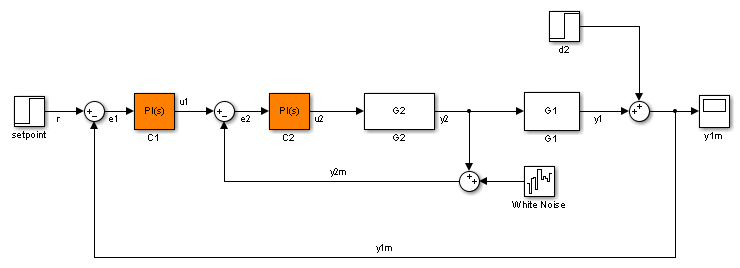### Open Model Linearizer for the Model

At the MATLAB® command line, open the Simulink model.

```mdl = 'scdcascade'; open_system(mdl)```

To open the Model Linearizer, in the Simulink model window, in the Apps gallery, click Model Linearizer.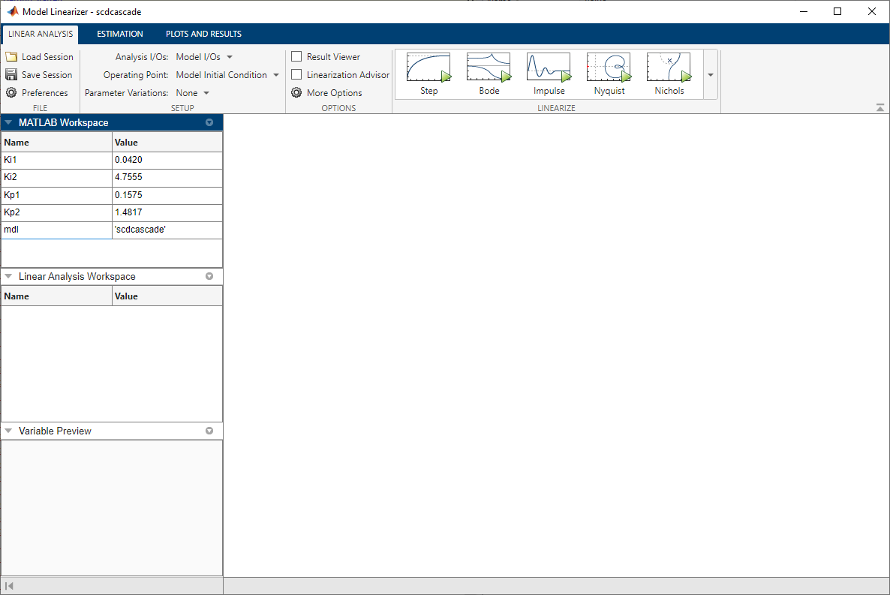### Vary the Inner-Loop Controller Gains

To analyze the behavior of the inner loop, very the gains of the inner-loop PI controller, `C2`. As you can see by inspecting the controller block, the proportional gain is the variable `Kp2`, and the integral gain is `Ki2`. Examine the performance of the inner loop for two different values of each of these gains.

In the Parameter Variations drop-down list, click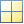`Select parameters to vary`.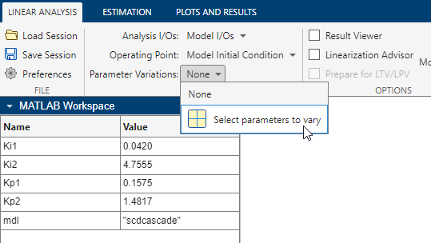The Parameter Variations tab opens. clickManage Parameters.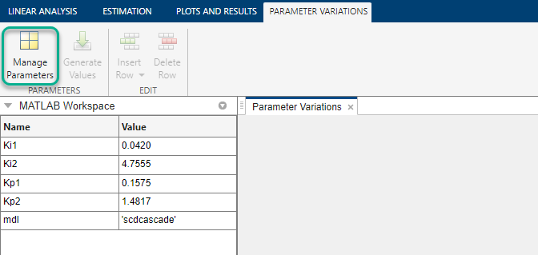In the Select model variables dialog box, check the parameters to vary, `Ki2` and `Kp2`.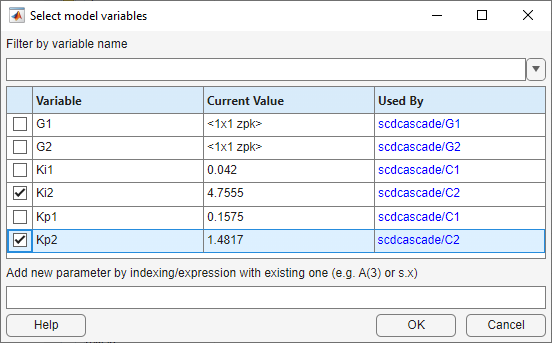The selected variables appear in the Parameter Variations table. Each column in the table corresponds to one of the selected variables. Each row in the table represents one `(Ki2,Kp2)` pair at which to linearize. These parameter-value combinations are called parameter samples. When you linearize, Model Linearizer computes as many linear models as there are parameter samples, or rows in the table.

Specify the parameter samples at which to linearize the model. For this example, specify four `(Ki2,Kp2)` pairs, `(Ki2,Kp2)` = (3.5,1), (3.5,2), (5,1), and (5,2). Enter these values in the table manually. To do so, select a row in the table. Then, select Insert Row > Insert Row Below twice.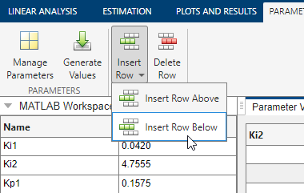Edit the values in the table as shown to specify the four `(Ki2,Kp2)` pairs.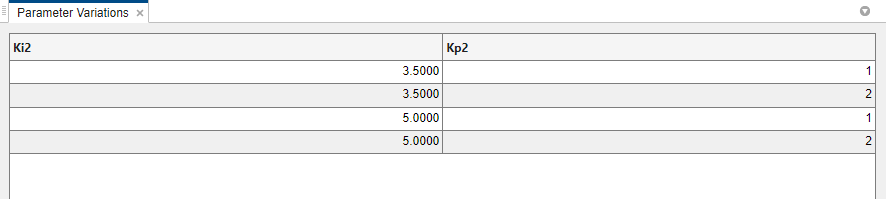### Tip

For more details about specifying parameter values, see Specify Parameter Samples for Batch Linearization

### Analyze the Inner Loop Closed-Loop Response

To analyze the inner-loop performance, extract a transfer function from the inner-loop input `u1` to the inner-plant output `y2`, computed with the outer loop open. To specify this I/O for linearization, in the Linear Analysis tab, in the Analysis I/Os drop-down list, select ```Create New Linearization I/Os```.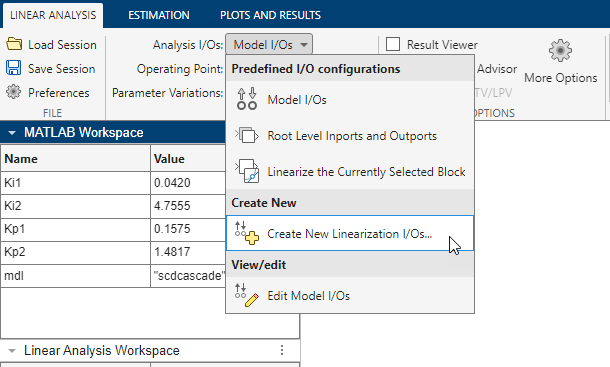Specify the I/O set by creating:

• An input perturbation point at `u1`

• An output measurement point at `y2`

• A loop break at `e1`

Name the I/O set by typing `InnerLoop` in the Variable name field of the Create linearization I/O set dialog box. The configuration of the dialog box is as shown.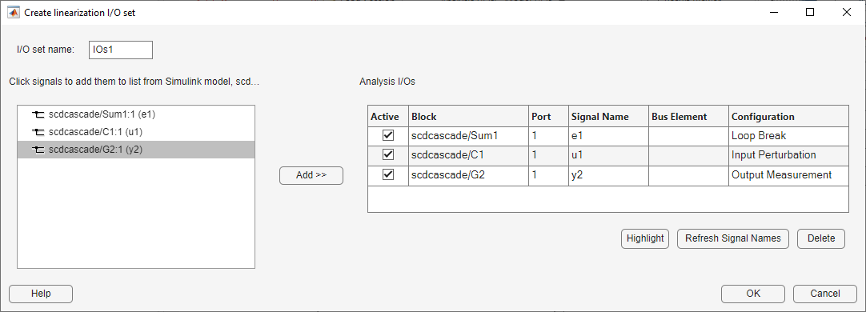### Tip

Click OK.

Now that you have specified the parameter variations and the analysis I/O set for the inner loop, linearize the model and examine a step response plot. Click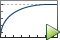Step.

Model Linearizer linearizes the model at each of the parameter samples you specified in the Parameter Variations table. A new variable, `linsys1`, appears in the Linear Analysis Workspace section of the Data Browser. This variable is an array of state-space (`ss`) models, one for each `(Ki2,Kp2)` pair. The plot shows the step responses of all the entries in `linsys1`. This plot gives you a sense of the range of step responses of the system in the operating ranges covered by the parameter grid.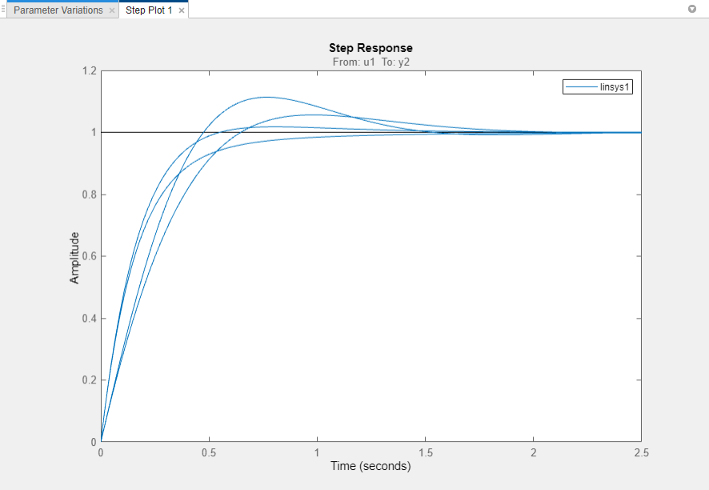### Vary the Outer-Loop Controller Gains

Examine the overall performance of the cascaded control system for varying values of the outer-loop controller, `C1`. To do so, vary the coefficients `Ki1` and `Kp1`, while keeping `Ki2` and `Kp2` fixed at the values specified in the model.

In the Parameter Variations tab, clickManage Parameters. Clear the `Ki2` and `Kp2` checkboxes, and check `Ki1` and `Kp1`. Click OK.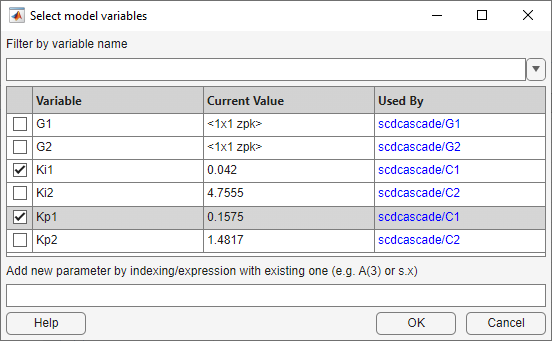Use Model Linearizer to generate parameter values automatically. ClickGenerate Values. In the Values column of the Generate Parameter Values table, enter an expression specifying the possible values for each parameter. For example, vary `Kp1` and `Ki1` by ± 50% of their nominal values, by entering expressions as shown.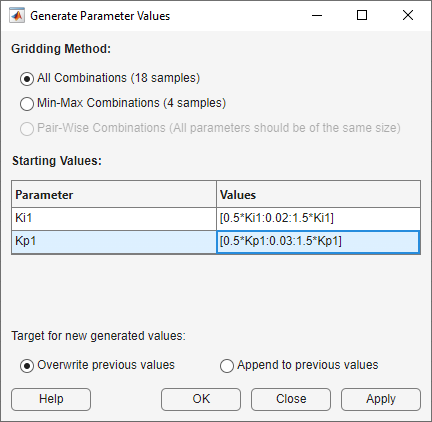The All Combinations gridding method generates a complete parameter grid of `(Kp1,Ki1)` pairs, to compute a linearization at all possible combinations of the specified values. ClickOverwrite to replace all values in the Parameter Variations table with the generated values.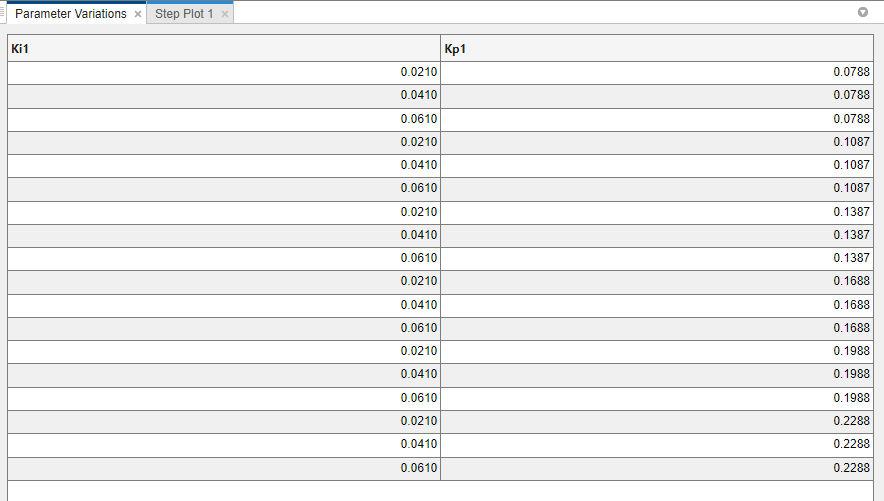Because you want to examine the overall closed-loop transfer function of the system, create a new linearization I/O set. In the Linear Analysis tab, in the Analysis I/Os drop-down list, select `Create New Linearization I/Os`. Configure `r` as an input perturbation point, and the system output `y1m` as an output measurement. Click OK.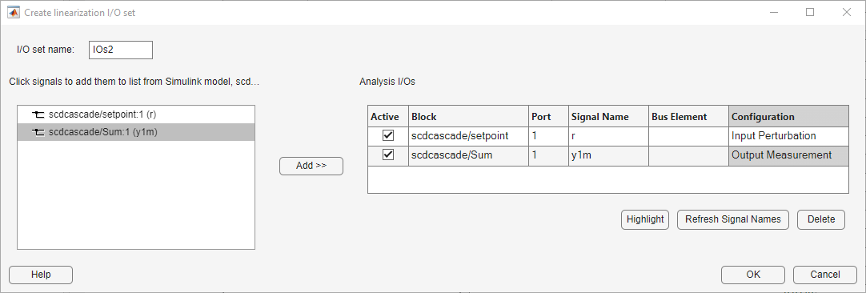Linearize the model with the parameter variations and examine the step response of the resulting models. ClickStep to linearize and generate a new plot for the new model array, `linsys2`.The step plot shows the responses of every model in the array. This plot gives you a sense of the range of step responses of the system in the operating ranges covered by the parameter grid.

### Note

Although the new plot reflects the new set of parameter variations, `Step Plot 1` and `linsys1` are unchanged. That plot and array still reflect the linearizations obtained with the inner-loop parameter variations.

### Further Analysis of Batch Linearization Results

The results of both batch linearizations, `linsys1` and `linsys2`, are arrays of state-space (`ss`) models. Use these arrays for further analysis in any of several ways:

Also see Validate Batch Linearization Results for information about validating linearization results in the MATLAB workspace.

## Related Topics